# Gaussian Process for Machine Learning¶

Examples concerning the sklearn.gaussian_process module.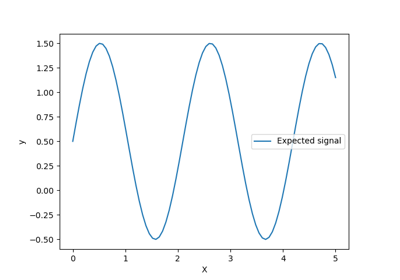Ability of Gaussian process regression (GPR) to estimate data noise-level

Ability of Gaussian process regression (GPR) to estimate data noise-level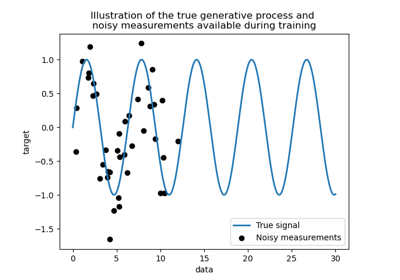Comparison of kernel ridge and Gaussian process regression

Comparison of kernel ridge and Gaussian process regression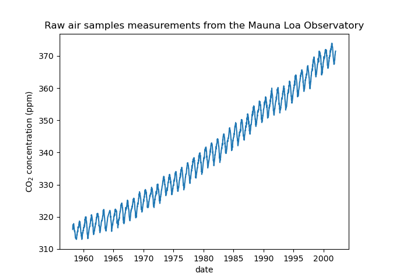Forecasting of CO2 level on Mona Loa dataset using Gaussian process regression (GPR)

Forecasting of CO2 level on Mona Loa dataset using Gaussian process regression (GPR)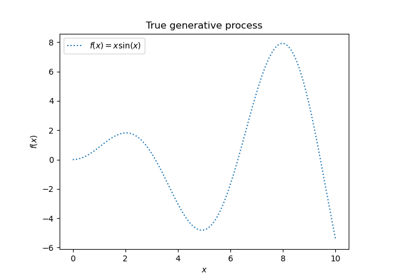Gaussian Processes regression: basic introductory example

Gaussian Processes regression: basic introductory example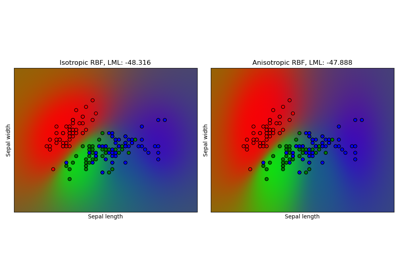Gaussian process classification (GPC) on iris dataset

Gaussian process classification (GPC) on iris dataset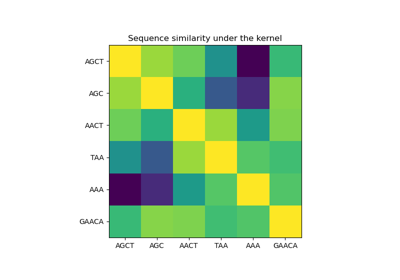Gaussian processes on discrete data structures

Gaussian processes on discrete data structures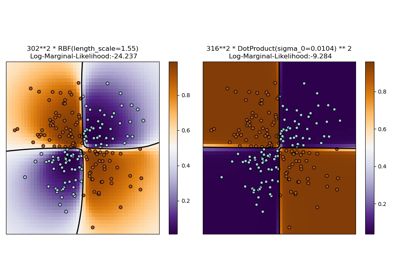Illustration of Gaussian process classification (GPC) on the XOR dataset

Illustration of Gaussian process classification (GPC) on the XOR dataset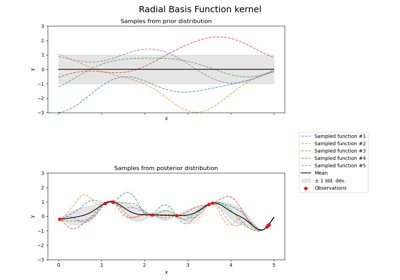Illustration of prior and posterior Gaussian process for different kernels

Illustration of prior and posterior Gaussian process for different kernels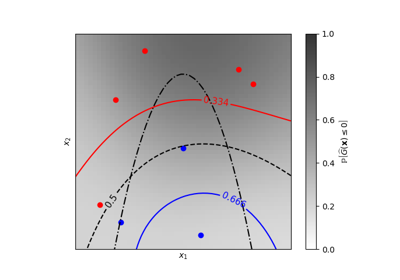Iso-probability lines for Gaussian Processes classification (GPC)

Iso-probability lines for Gaussian Processes classification (GPC)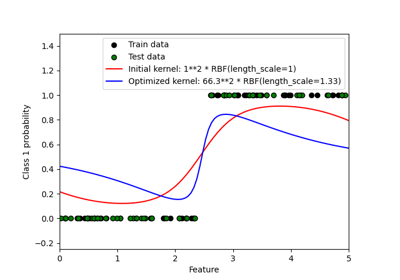Probabilistic predictions with Gaussian process classification (GPC)

Probabilistic predictions with Gaussian process classification (GPC)